# RD Sharma Solutions for Class 8 Maths Chapter 1 - Rational Numbers Exercise 1.5

In Exercise 1.5 of RD Sharma Class 8 Maths Chapter 1 Rational numbers, we shall discuss problems based on the multiplication of rational numbers. Students can refer and download RD Sharma Class 8 Solutions for Maths Exercise 1.5 from the links provided below. Problems are solved by our experts in order to help students in learning the concepts with ease from the textbook.

## Download PDF of RD Sharma Solutions for Class 8 Maths Exercise 1.5 Chapter 1 Rational Numbers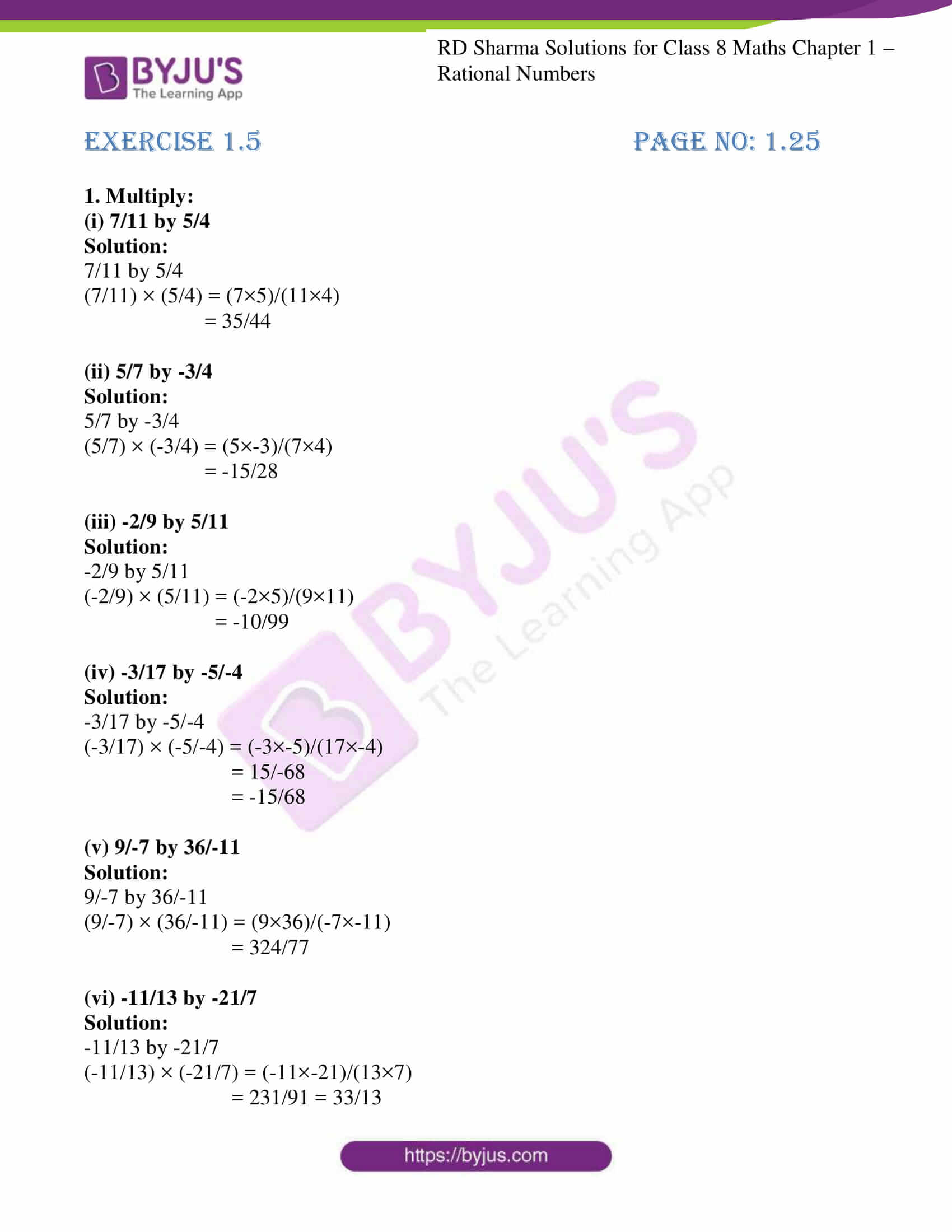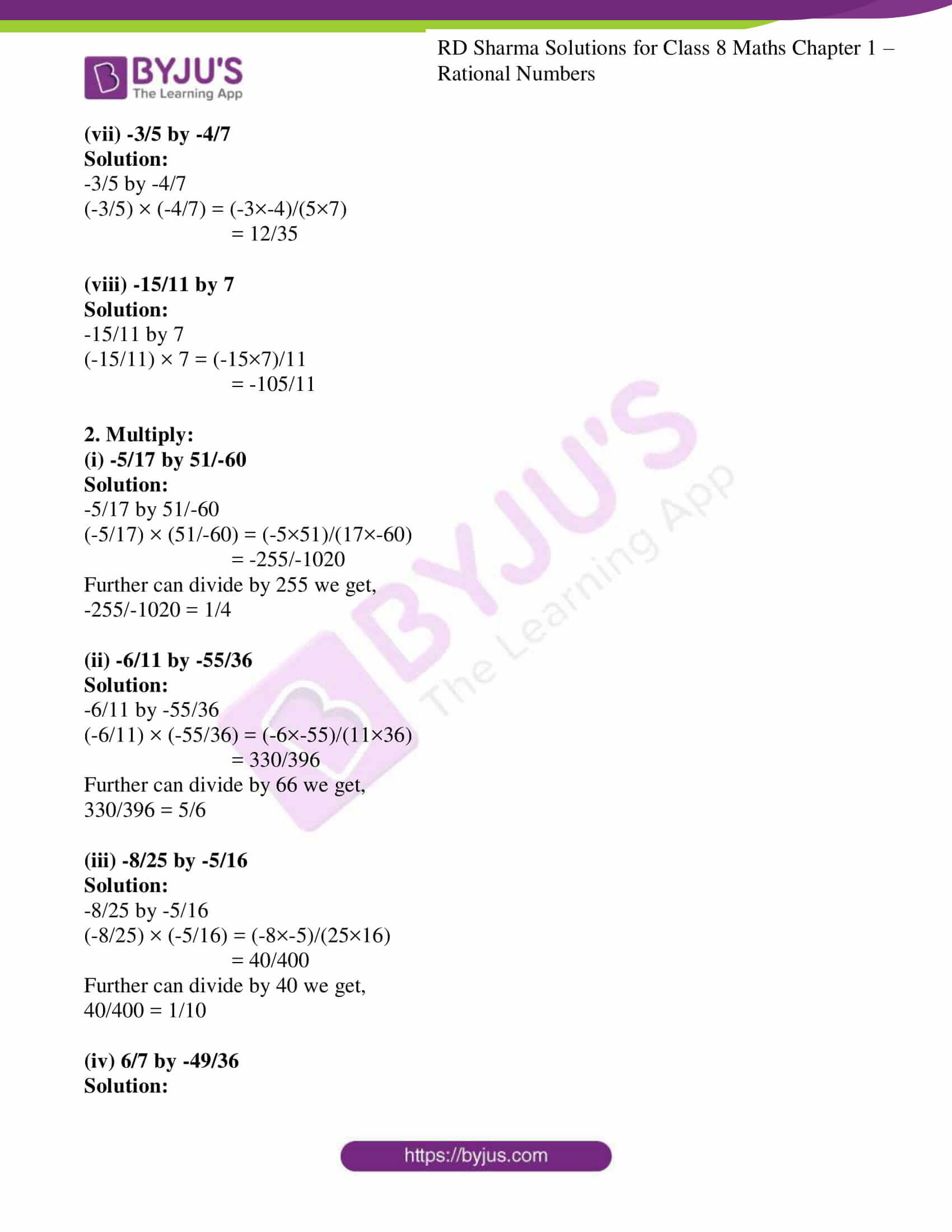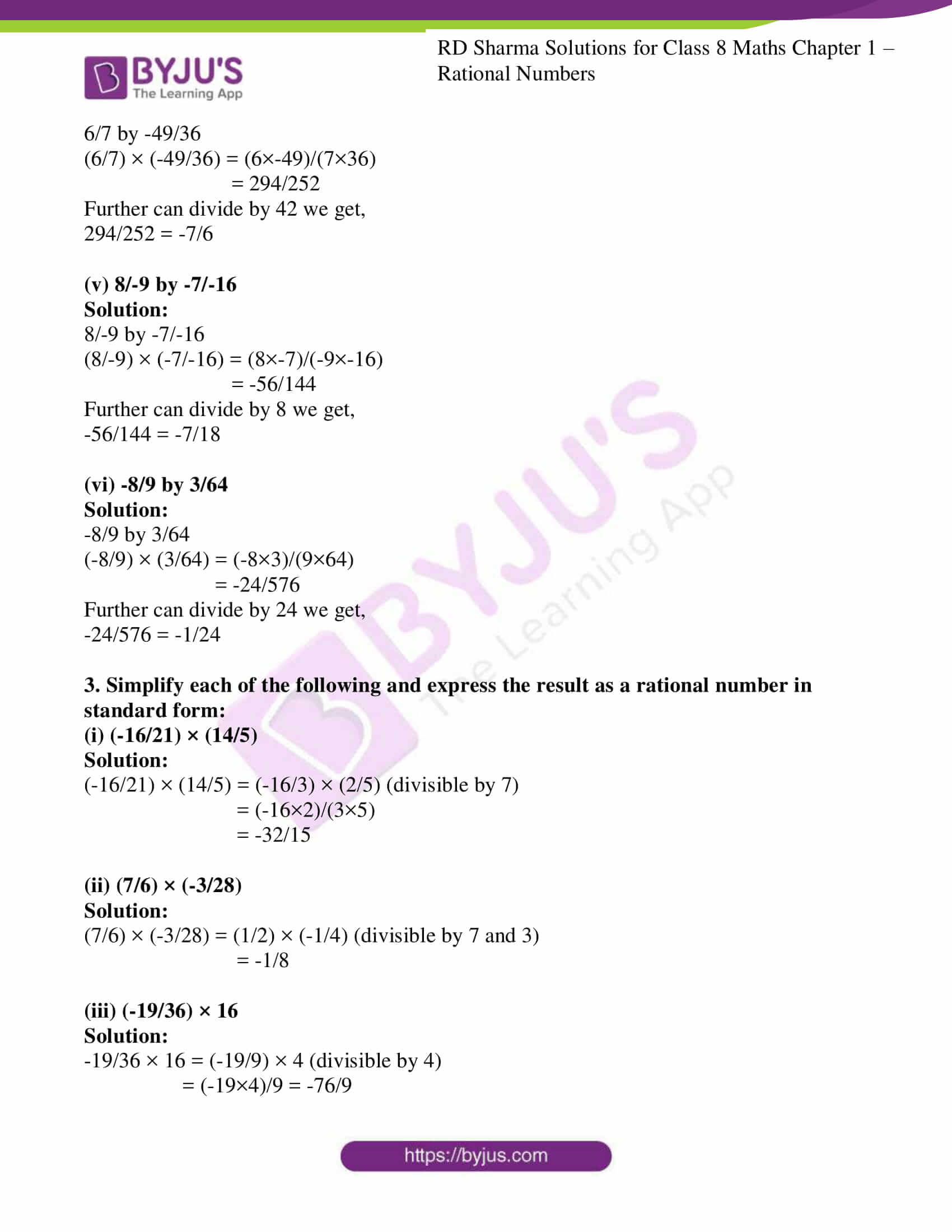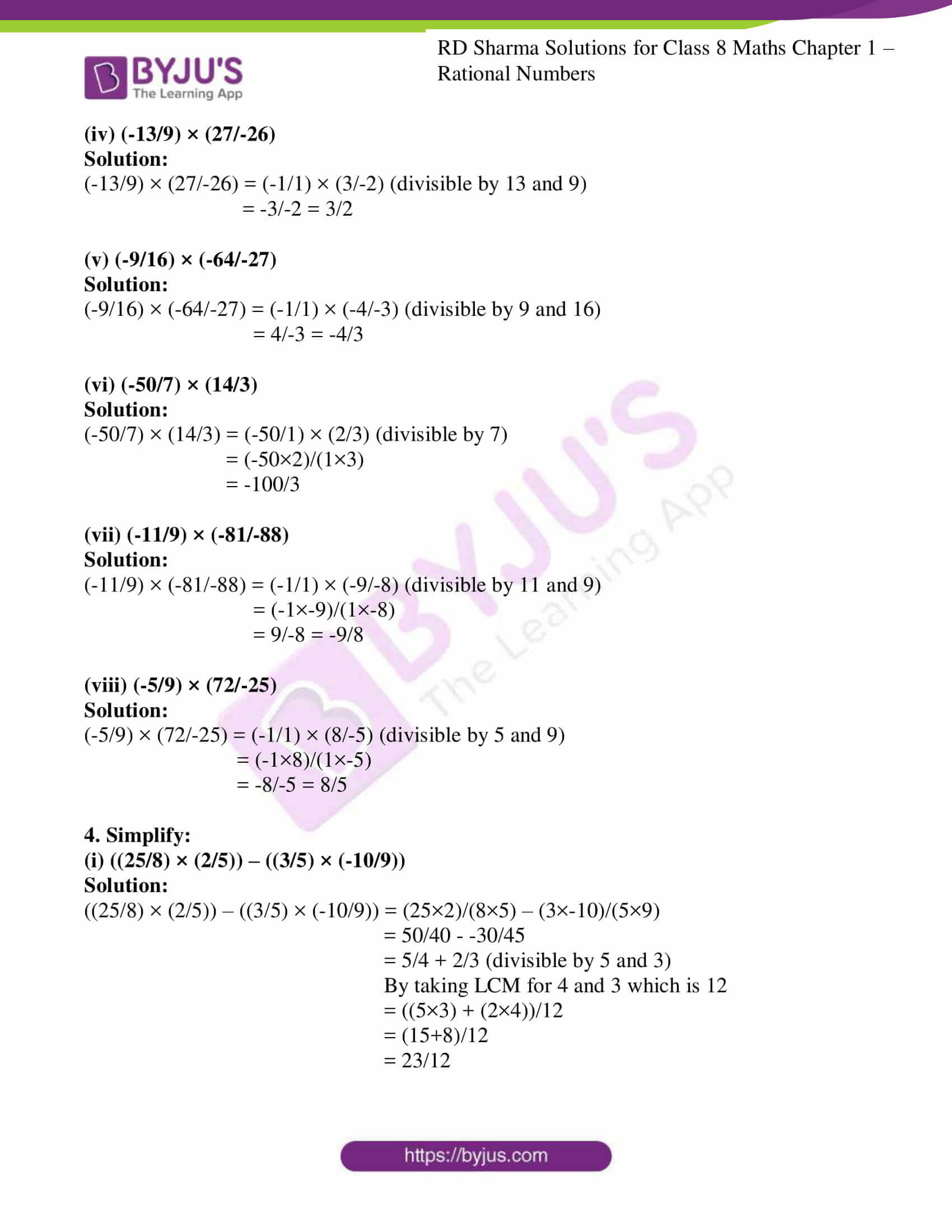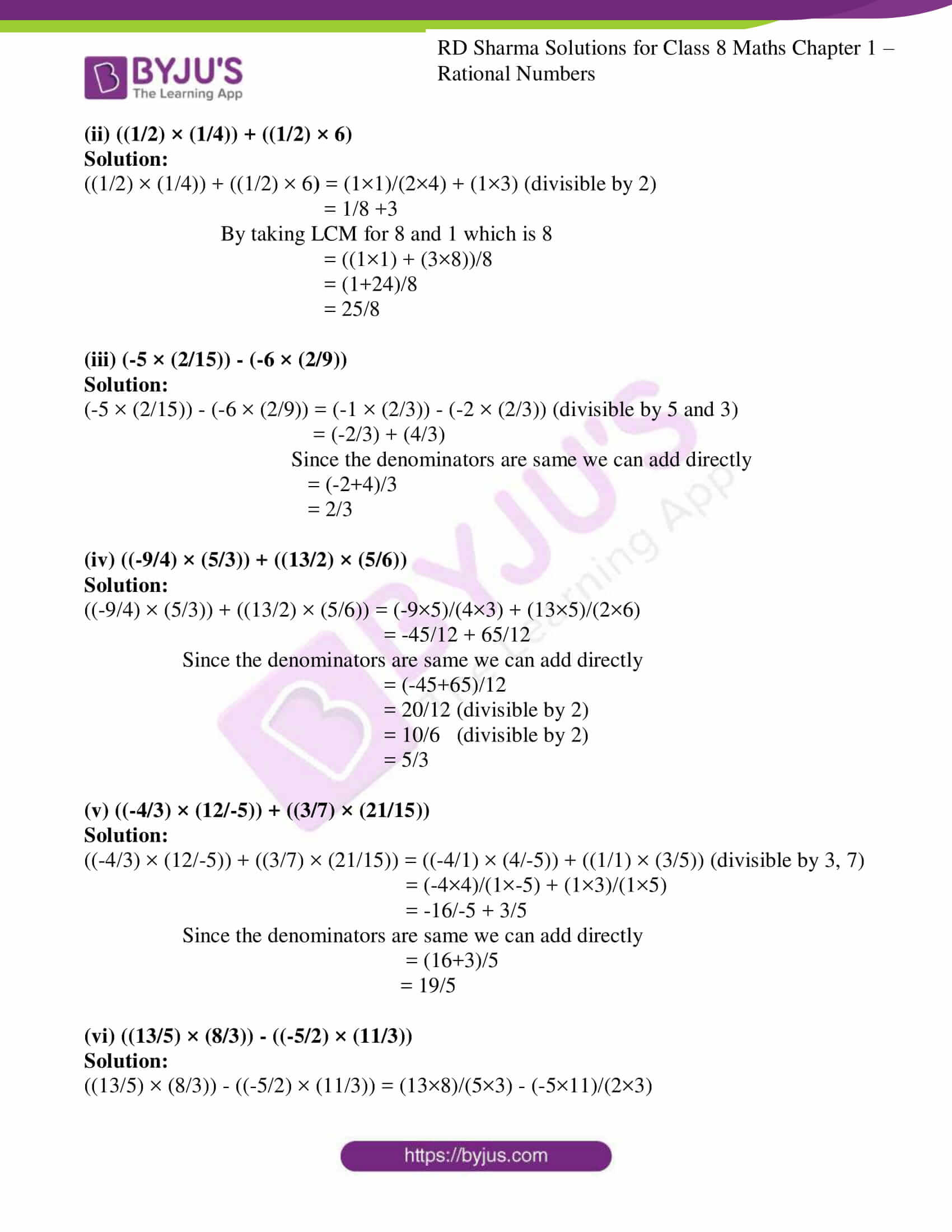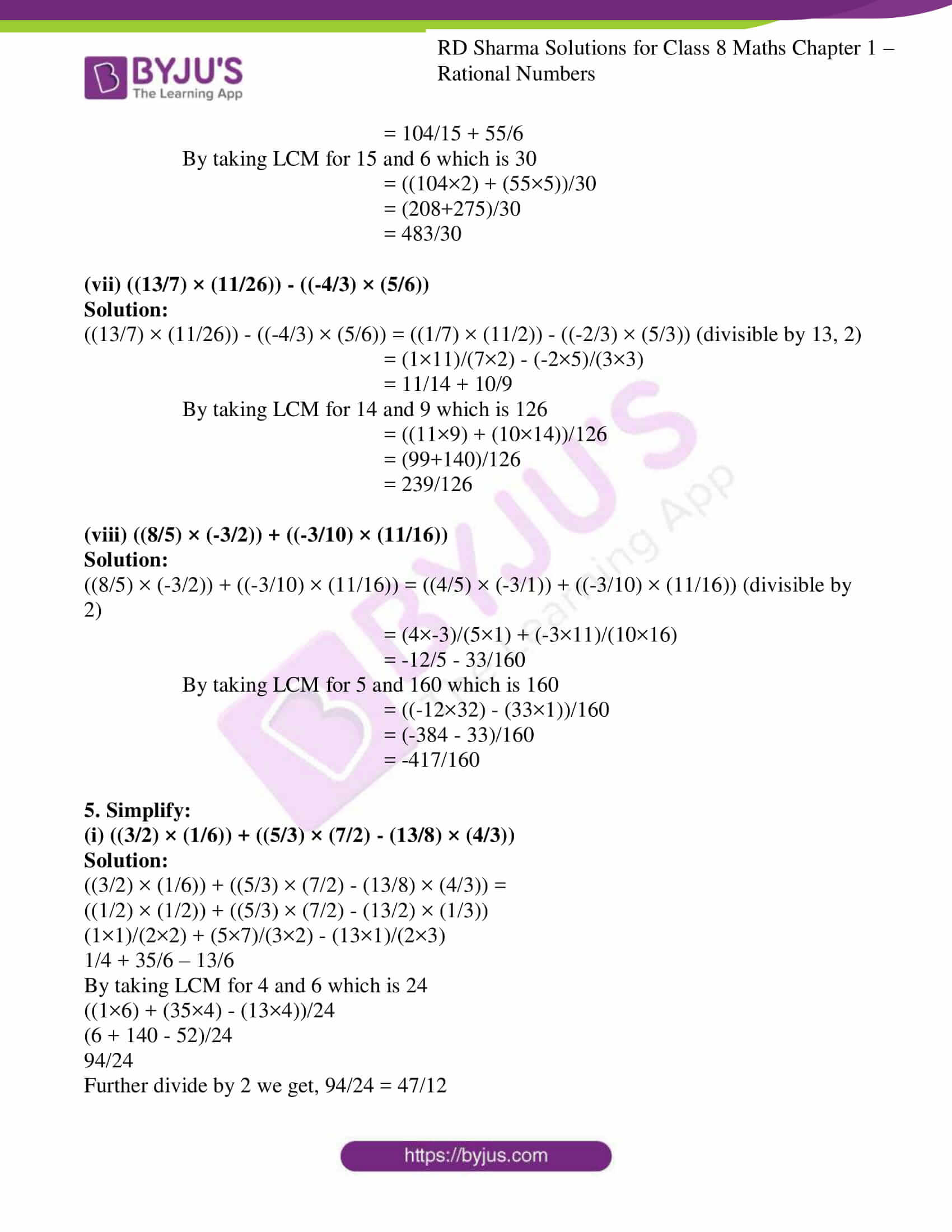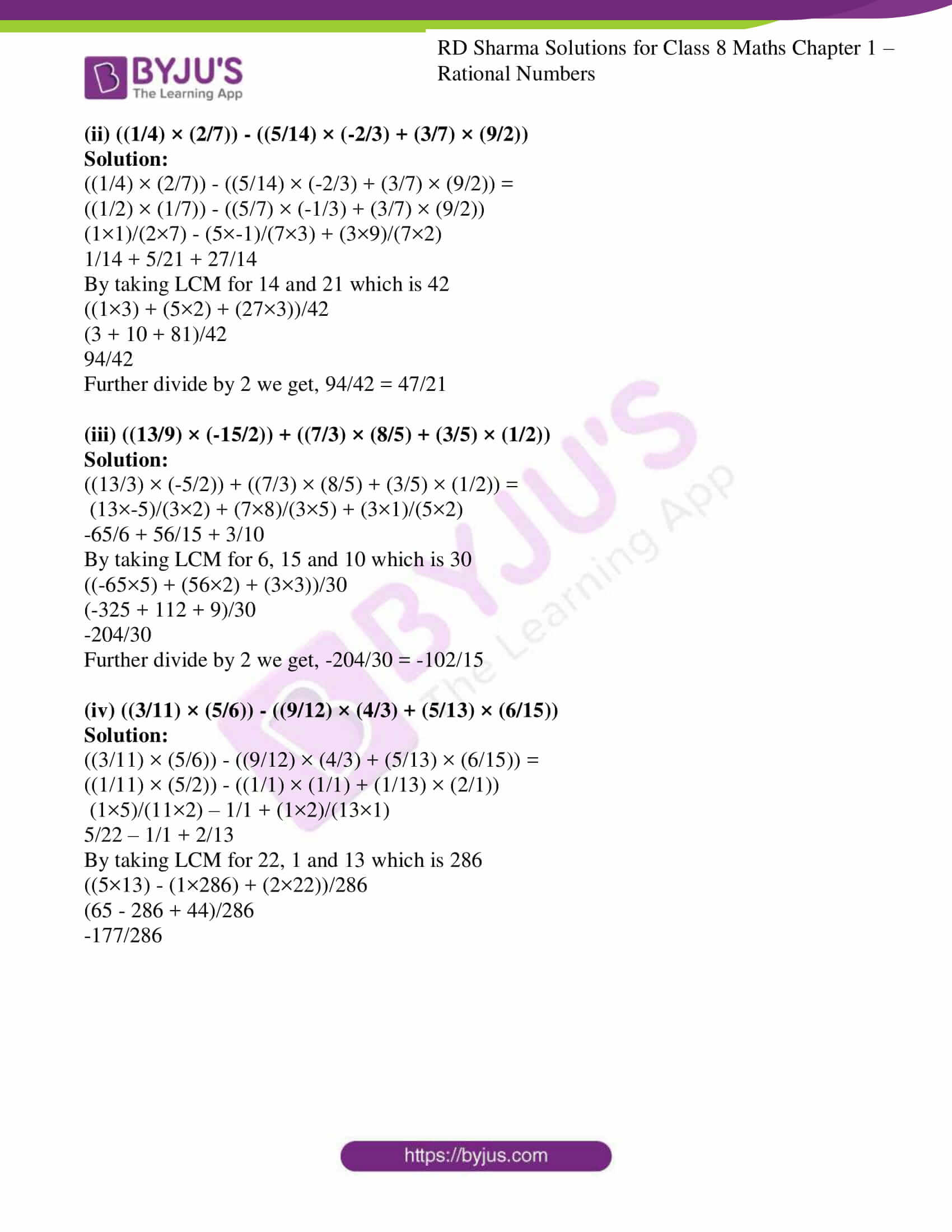### Access Answers to RD Sharma Solutions for Class 8 Maths Exercise 1.5 Chapter 1 Rational Numbers

1. Multiply:

(i) 7/11 by 5/4

Solution:

7/11 by 5/4

(7/11) × (5/4) = (7×5)/(11×4)

= 35/44

(ii) 5/7 by -3/4

Solution:

5/7 by -3/4

(5/7) × (-3/4) = (5×-3)/(7×4)

= -15/28

(iii) -2/9 by 5/11

Solution:

-2/9 by 5/11

(-2/9) × (5/11) = (-2×5)/(9×11)

= -10/99

(iv) -3/17 by -5/-4

Solution:

-3/17 by -5/-4

(-3/17) × (-5/-4) = (-3×-5)/(17×-4)

= 15/-68

= -15/68

(v) 9/-7 by 36/-11

Solution:

9/-7 by 36/-11

(9/-7) × (36/-11) = (9×36)/(-7×-11)

(vi) -11/13 by -21/7

Solution:

-11/13 by -21/7

(-11/13) × (-21/7) = (-11×-21)/(13×7)

= 231/91 = 33/13

(vii) -3/5 by -4/7

Solution:

-3/5 by -4/7

(-3/5) × (-4/7) = (-3×-4)/(5×7)

= 12/35

(viii) -15/11 by 7

Solution:

-15/11 by 7

(-15/11) × 7 = (-15×7)/11

= -105/11

2. Multiply:

(i) -5/17 by 51/-60

Solution:

-5/17 by 51/-60

(-5/17) × (51/-60) = (-5×51)/(17×-60)

= -255/-1020

Further can divide by 255 we get,

-255/-1020 = 1/4

(ii) -6/11 by -55/36

Solution:

-6/11 by -55/36

(-6/11) × (-55/36) = (-6×-55)/(11×36)

= 330/396

Further can divide by 66 we get,

330/396 = 5/6

(iii) -8/25 by -5/16

Solution:

-8/25 by -5/16

(-8/25) × (-5/16) = (-8×-5)/(25×16)

= 40/400

Further can divide by 40 we get,

40/400 = 1/10

(iv) 6/7 by -49/36

Solution:

6/7 by -49/36

(6/7) × (-49/36) = (6×-49)/(7×36)

= 294/252

Further can divide by 42 we get,

294/252 = -7/6

(v) 8/-9 by -7/-16

Solution:

8/-9 by -7/-16

(8/-9) × (-7/-16) = (8×-7)/(-9×-16)

= -56/144

Further can divide by 8 we get,

-56/144 = -7/18

(vi) -8/9 by 3/64

Solution:

-8/9 by 3/64

(-8/9) × (3/64) = (-8×3)/(9×64)

= -24/576

Further can divide by 24 we get,

-24/576 = -1/24

3. Simplify each of the following and express the result as a rational number in standard form:

(i) (-16/21) × (14/5)

Solution:

(-16/21) × (14/5) = (-16/3) × (2/5) (divisible by 7)

= (-16×2)/(3×5)

= -32/15

(ii) (7/6) × (-3/28)

Solution:

(7/6) × (-3/28) = (1/2) × (-1/4) (divisible by 7 and 3)

= -1/8

(iii) (-19/36) × 16

Solution:

-19/36 × 16 = (-19/9) × 4 (divisible by 4)

= (-19×4)/9 = -76/9

(iv) (-13/9) × (27/-26)

Solution:

(-13/9) × (27/-26) = (-1/1) × (3/-2) (divisible by 13 and 9)

= -3/-2 = 3/2

(v) (-9/16) × (-64/-27)

Solution:

(-9/16) × (-64/-27) = (-1/1) × (-4/-3) (divisible by 9 and 16)

= 4/-3 = -4/3

(vi) (-50/7) × (14/3)

Solution:

(-50/7) × (14/3) = (-50/1) × (2/3) (divisible by 7)

= (-50×2)/(1×3)

= -100/3

(vii) (-11/9) × (-81/-88)

Solution:

(-11/9) × (-81/-88) = (-1/1) × (-9/-8) (divisible by 11 and 9)

= (-1×-9)/(1×-8)

= 9/-8 = -9/8

(viii) (-5/9) × (72/-25)

Solution:

(-5/9) × (72/-25) = (-1/1) × (8/-5) (divisible by 5 and 9)

= (-1×8)/(1×-5)

= -8/-5 = 8/5

4. Simplify:

(i) ((25/8) × (2/5)) – ((3/5) × (-10/9))

Solution:

((25/8) × (2/5)) – ((3/5) × (-10/9)) = (25×2)/(8×5) – (3×-10)/(5×9)

= 50/40 – -30/45

= 5/4 + 2/3 (divisible by 5 and 3)

By taking LCM for 4 and 3 which is 12

= ((5×3) + (2×4))/12

= (15+8)/12

= 23/12

(ii) ((1/2) × (1/4)) + ((1/2) × 6)

Solution:

((1/2) × (1/4)) + ((1/2) × 6) = (1×1)/(2×4) + (1×3) (divisible by 2)

= 1/8 +3

By taking LCM for 8 and 1 which is 8

= ((1×1) + (3×8))/8

= (1+24)/8

= 25/8

(iii) (-5 × (2/15)) – (-6 × (2/9))

Solution:

(-5 × (2/15)) – (-6 × (2/9)) = (-1 × (2/3)) – (-2 × (2/3)) (divisible by 5 and 3)

= (-2/3) + (4/3)

Since the denominators are same we can add directly

= (-2+4)/3

= 2/3

(iv) ((-9/4) × (5/3)) + ((13/2) × (5/6))

Solution:

((-9/4) × (5/3)) + ((13/2) × (5/6)) = (-9×5)/(4×3) + (13×5)/(2×6)

= -45/12 + 65/12

Since the denominators are same we can add directly

= (-45+65)/12

= 20/12 (divisible by 2)

= 10/6 (divisible by 2)

= 5/3

(v) ((-4/3) × (12/-5)) + ((3/7) × (21/15))

Solution:

((-4/3) × (12/-5)) + ((3/7) × (21/15)) = ((-4/1) × (4/-5)) + ((1/1) × (3/5)) (divisible by 3, 7)

= (-4×4)/(1×-5) + (1×3)/(1×5)

= -16/-5 + 3/5

Since the denominators are same we can add directly

= (16+3)/5

= 19/5

(vi) ((13/5) × (8/3)) – ((-5/2) × (11/3))

Solution:

((13/5) × (8/3)) – ((-5/2) × (11/3)) = (13×8)/(5×3) – (-5×11)/(2×3)

= 104/15 + 55/6

By taking LCM for 15 and 6 which is 30

= ((104×2) + (55×5))/30

= (208+275)/30

= 483/30

(vii) ((13/7) × (11/26)) – ((-4/3) × (5/6))

Solution:

((13/7) × (11/26)) – ((-4/3) × (5/6)) = ((1/7) × (11/2)) – ((-2/3) × (5/3)) (divisible by 13, 2)

= (1×11)/(7×2) – (-2×5)/(3×3)

= 11/14 + 10/9

By taking LCM for 14 and 9 which is 126

= ((11×9) + (10×14))/126

= (99+140)/126

= 239/126

(viii) ((8/5) × (-3/2)) + ((-3/10) × (11/16))

Solution:

((8/5) × (-3/2)) + ((-3/10) × (11/16)) = ((4/5) × (-3/1)) + ((-3/10) × (11/16)) (divisible by 2)

= (4×-3)/(5×1) + (-3×11)/(10×16)

= -12/5 – 33/160

By taking LCM for 5 and 160 which is 160

= ((-12×32) – (33×1))/160

= (-384 – 33)/160

= -417/160

5. Simplify:

(i) ((3/2) × (1/6)) + ((5/3) × (7/2) – (13/8) × (4/3))

Solution:

((3/2) × (1/6)) + ((5/3) × (7/2) – (13/8) × (4/3)) =

((1/2) × (1/2)) + ((5/3) × (7/2) – (13/2) × (1/3))

(1×1)/(2×2) + (5×7)/(3×2) – (13×1)/(2×3)

1/4 + 35/6 – 13/6

By taking LCM for 4 and 6 which is 24

((1×6) + (35×4) – (13×4))/24

(6 + 140 – 52)/24

94/24

Further divide by 2 we get, 94/24 = 47/12

(ii) ((1/4) × (2/7)) – ((5/14) × (-2/3) + (3/7) × (9/2))

Solution:

((1/4) × (2/7)) – ((5/14) × (-2/3) + (3/7) × (9/2)) =

((1/2) × (1/7)) – ((5/7) × (-1/3) + (3/7) × (9/2))

(1×1)/(2×7) – (5×-1)/(7×3) + (3×9)/(7×2)

1/14 + 5/21 + 27/14

By taking LCM for 14 and 21 which is 42

((1×3) + (5×2) + (27×3))/42

(3 + 10 + 81)/42

94/42

Further divide by 2 we get, 94/42 = 47/21

(iii) ((13/9) × (-15/2)) + ((7/3) × (8/5) + (3/5) × (1/2))

Solution:

((13/3) × (-5/2)) + ((7/3) × (8/5) + (3/5) × (1/2)) =

(13×-5)/(3×2) + (7×8)/(3×5) + (3×1)/(5×2)

-65/6 + 56/15 + 3/10

By taking LCM for 6, 15 and 10 which is 30

((-65×5) + (56×2) + (3×3))/30

(-325 + 112 + 9)/30

-204/30

Further divide by 2 we get, -204/30 = -102/15

(iv) ((3/11) × (5/6)) – ((9/12) × (4/3) + (5/13) × (6/15))

Solution:

((3/11) × (5/6)) – ((9/12) × (4/3) + (5/13) × (6/15)) =

((1/11) × (5/2)) – ((1/1) × (1/1) + (1/13) × (2/1))

(1×5)/(11×2) – 1/1 + (1×2)/(13×1)

5/22 – 1/1 + 2/13

By taking LCM for 22, 1 and 13 which is 286

((5×13) – (1×286) + (2×22))/286

(65 – 286 + 44)/286

-177/286

## RD Sharma Solutions for Class 8 Maths Exercise 1.5 Chapter 1 Rational Numbers

Class 8 Maths Chapter 1 Rational Numbers Exercise 1.5 is based on the multiplication of rational numbers. These solutions are prepared by experienced faculty in accordance with CBSE syllabus for 8th standard. The exercise-wise solutions are explained in a simple and easily understandable language which helps students excel in their exams. Download free RD Sharma Solutions Chapter 1 in PDF format which provides answers to all the questions.